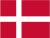### Dish Cloth 55

The pattern wasn't very distinct in dish cloth 52, so I have tried to  double it.

Cast on 64 stitches and knit 4 rows and then pattern until the cloth has the desired length. Knit 4 rows and bind off.

Pattern:

1.row: 2 k, 2 p, 2 k, 2 p, 6 k, 2 p
2.row: 2 k, 6 p, 2 k, 2 p, 2 k, 2 p
3.row: 6 k, 4 p, 2 k, 4 p
4.row: 4 k, 2 p, 4 k, 6 p
5.row: 6 k, 10 p
6.row: 10 k, 6 p
7.row: 2 p, 2 k, 2 p, 2 k, 6 p, 2 k
8.row: 2 p, 6 k, 2 p, 2 k, 2 p, 2 k
9.row: 6 p, 4 k, 2 p, 4 k
10.row: 4 p, 2 k, 4 p, 6 k
11.row: 1 k, 4 p, 11 k
12.row: 11 p, 4 k, 1 p

 X X X X 12 X X X X 11 X X X X X X X X 10 X X X X X X X X 9 X X X X X X X X X X 8 X X X X X X X X X X 7 X X X X X X X X X X 6 X X X X X X X X X X 5 X X X X X X X X 4 X X X X X X X X 3 X X X X X X 2 X X X X X X 1
You have to remember that all uneven rows start in the right side of the diagram and X=purl and ▢= knit. All equal rows start in the left side of the diagram and X=knit and ▢=purl.Danish